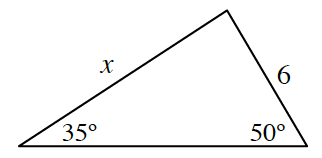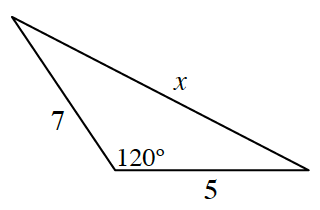### Home > PC3 > Chapter 11 > Lesson 11.1.2 > Problem11-32

11-32.

Solve for the value of $x$ for each triangle.

1.Use the Law of Sines.

1.Use the Law of Cosines.

1. What is the area of the triangle in part (a)?

Determine the measure of the unlabeled angle.
Then the formula $\text{area} = 0.5ab{\sin(θ)}$ can be used to calculate the area.